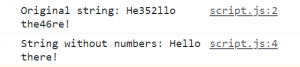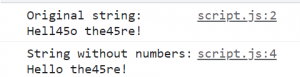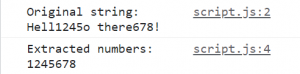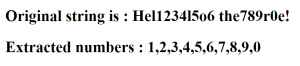# How to extract numbers from a string in JavaScript?

In this tutorial, we’ll look at how to extract numbers from a string in JavaScript. We’ll be working on 2 solutions, one that extracts the string, without numbers, into a variable, so you can use it later.

The other solution will extract just the numbers as an array, so again, you can use it later.

Let’s look at different ways of approaching both the solutions now.

## HTML Skeleton

To start with, I’ve opened up a new project in my Visual Studio Code editor, and I’ve created a standard HTML skeleton as follows:

```<!DOCTYPE html>
<html lang="en">
<meta charset="UTF-8">
<meta http-equiv="X-UA-Compatible" content="IE=edge">
<meta name="viewport" content="width=device-width, initial-scale=1.0">
<title>JavaScript practice</title>
<body>

<script src="script.js"></script>
</body>
</html>
```

As you can see, I’ve created a script.js file, and connected that to my HTML file as well. Now, let’s get started!

## The String Replace Method to remove the numbers

With all these examples, we’re going to be using regular expressions. Here, to start with, we’re going to give a condition that says “Replace every digit (0 to 9) with an empty space. This will essentially remove those digits from the string without a trace.

In regular expressions, you can use \d to denote digits, and as shown in the example below, by using the ‘g’ flag, which denotes ‘global’, we’re instructing the browser to remove every single digit it comes across in the string.

If you don’t use the global flag, then the browser would just remove the first digit it comes across and leaves the rest as it is.
With ‘replace’ method and \d which denotes digits:

```let str = prompt("Enter the string with numbers");
console.log(`Original string: \${str}`);
str = str.replace(/\d/g,'');
console.log(`String without numbers: \${str}`);
```

Now, let’s run this code and see what happens. I’ve installed a ‘live server’ plugin in Visual studio code to run a development server (localhost) from my system, and I use that to check my code during development.

Since I’ve asked for a user prompt, the minute I open my server, I get a prompt that asks for a string with numbers. I’ve entered the same, as shown below:Press Ok, and you’ll get the following:As you can see, I get the original string first, and then the string without numbers, as I wanted.

So that’s it! That’s how you remove numbers from a string. You can use this code in instances where you find yourself with a piece of text with junk numbers, or something like that.

## More ways of doing the same

Now, why don’t we look at other ways of arriving at the same output?

Instead of \d, we can use [0-9] to denote numbers/digits, and get the same results:

`str = str.replace(/[0-9]/g,'');`

We can use the ‘+’ symbol instead of the global flag too. ‘+’ denotes ‘one or more’, and it means, remove 1 or more instances of any of the digits from the string.

`str = str.replace(/\d+/,'');`
`str = str.replace(/[0-9]+/,'');`

But unfortunately, the ‘+’ symbol won’t work for multi-word strings, as you can see below.The numbers in the first word were removed, but those in the second word remains the same. So, again, you have no choice but to use the global flag to make it work across multiple words.

## Extracting the numbers

If we would like to extract the numbers and display them instead, use \D, which denotes the non-digit characters:

`str = str.replace(/\D/g,'');`

Instead, you can use the ^ (carot) symbol while denoting the digits, which again refers to ‘non’ digits. ^ means ‘not’ in this instance.

`str = str.replace(/[^0-9]/g,'');`

Run either of the above lines of code, and you’ll get the following output:Also, to extract the numbers, we can use the ‘match’ method:

`let nums = str.match(/\d/g);`

The result of this method will be an object though. Now, how do we display it in the DOM? Let’s create a bunch of h2 tags, give them ids, and call them from our script file, as follows:

```<h2 id="str"></h2>
<h2 id="num"></h2>
```

Then, let’s change the innerText of those h2 tags to separately get the original string, and the extracted numbers:

```let str = prompt("Enter the string with numbers");
let strEl = document.getElementById('str');
strEl.innerText = `Original string is : \${str}`;
let nums = str.match(/\d/g);
let numEl = document.getElementById('num');
numEl.innerText = `Extracted numbers : \${nums}`;
```

When you run the above, you’ll get the following:As you can see, this is a piece of string that you can manipulate as you like.

So, that’s it! Those are the different ways of extracting/removing numbers from a string.